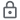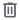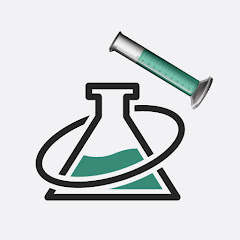# Chemical Equation Balancer

50K+Everyone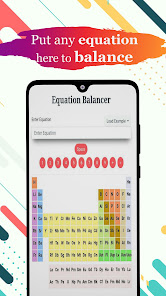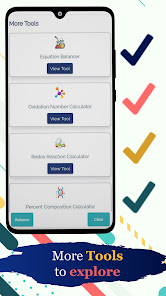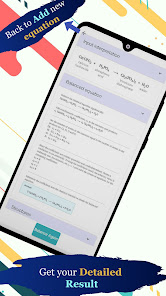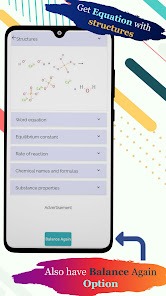Chemical Equation Balancer to easily measure chemistry formulas. It gives you the finest experience to solve problems of equations and chemical reactions with a chemistry calculator.

This Chemical Equation Balance App is a smart tool which works accurately as a chemical balancer. Students of any level and class can use this chemistry solver app because we have designed it with an easy to use interface for balancing chemical equation. This app contains all chemistry equations, so you can easily measure and balance equation of chemistry with this Chemical Equation Balancing Calculator.

Good thing of this chemistry equation app is it also contains a periodic table. So that, you’ll be able to find the Chemical Formulas and Chemistry Equations in no time by using this Chemical Equation Balancer and chem calculator. We sure it will be your next favourite app as Chemical Balancer.

How to use chemistry calculator
This Chemical Equation Balance App enables you to solve equations in only a few seconds. You only need to write your desired equation and tap the balance button. Then this Chemical Equation Solver process on your query, solve equation and reactions to show accurate results in no time. You can also copy and paste equations or results of your balanced equation practice by solving Chemistry Equations and formulas with this calculator.

Features of Chemical Equation Balancer
- Small size chemistry calculator.
- Smooth Chemical Balancer App.
- Very easy to use.
- All chemical formulas.
- Solve chemistry equations.
- Periodic table.
- Balancing chemicals calculator for all.
- Useful app for students of chemistry.
- Easy Balancing Chemical Equation.
- Simple chemistry tool.
- One second for balancing the Chemical Reaction.
- Quick results of the chemistry balancer.

Remember that the Chemical Equation Balance App does not contain only the facility of equation balance. It also comprises all chemistry formulas and equations to solve and provide you the right answer by measuring equation problems. It is also a complete Chemical Equations Solver.

Purpose to making this balancing chemical calculator is to provide you with a smooth and easy to use interface and experience of Chemistry eEuation Balancing App. No need to search here and there for different formulas when you have this all in one learning app which contains all chemistry formulas and equations. Just insert the equation into the box and see the accurate result of the balanced equation by using this easy and perfect working Equation Balancer. For your ease, we have added a complete formula and equations of chemistry. Just choose any equation from them and after solving equations by this calculator, you’ll get the solution of your desired Chemical Equation.

If you are looking for easy to use and good Chemical Equation Balancer? This chemistry equation balancing app is for you. Now, you will not forget the formula of equations with the help of the periodic table of this chemistry balancer. Because it is our aim to let you easily learn and understand chemistry formulas and balance equation.

This is a very helpful chemical equation balancer. Use this simple chemistry tool for Balancing Chemical Equations and measure reactions of formulas in no time. We hope you’ll love this chemistry calculator and let us know your views and valuable words about this app to make it better to solve equations for you.

More Chemistry Calculators
This balancing chemical equation app also lets you to use other chemistry calculators like:
- Oxidation Number Calculator
- Redox Reaction Calculator
- Percent Composition Calculator
- Percent Yield Calculator
- Theoretical Yield Calculator
- Molecular Weight Calculator
- Titration Calculator
- Atomic Mass Calculator
Updated on
Jun 19, 2021

## Data safety

Safety starts with understanding how developers collect and share your data. Data privacy and security practices may vary based on your use, region, and age. The developer provided this information and may update it over time.This app may share these data types with third parties
LocationNo data collected